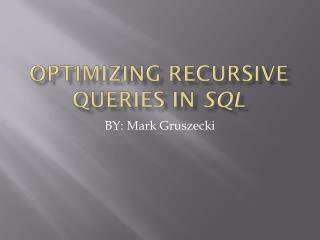# Optimizing recursive queries in sql - PowerPoint PPT PresentationDownload PresentationOptimizing recursive queries in sql

Optimizing recursive queries in sqlDownload Presentation## Optimizing recursive queries in sql

- - - - - - - - - - - - - - - - - - - - - - - - - - - E N D - - - - - - - - - - - - - - - - - - - - - - - - - - -
##### Presentation Transcript

1. Optimizing recursive queries in sql BY: Mark Gruszecki

2. Overview • What is a Recursive Query? • Definition(s) and Algorithm(s) • Optimization Techniques • Practical Issues • Impact of each Optimization • Conclusion

3. In order to understand a Recursive Query, you must first understand a Recursive Query… CREATE RECURSIVE VIEW R(d, i, j, v) AS ( SELECT 1, i, j, v FROM T UNION ALL SELECT d+1, R.i, T.j, R.v + T.v FROM R JOIN T ON R.j = T.i WHERE d < 8);

4. What is a Recursive Query used for? • Any kind of Hierarchical Data is more easily accessed using a Recursive Query. • For example, displaying Employees in an orginizational chart.

5. Definition(s) and Algorithm(s) • A base table T is defined as T(i, j, v) with primary key (i, j) and v representing a numerical value. • Define the result table from a recursive query by R(d, i, j, v) with primary key (d, i, j). • The queries of interest are of the form • R[k] = T  T  T  …  T where  is the recursive query. • Let G = (V, E) be a directed graph with n vertices and m edges. • The algorithm to evaluate optimizations is: • R =

6. Optimization Techniques • Early evaluation of row select conditions. • Deleting Duplicate Rows. • Indexing base and result tables for efficient joins.

7. Early Evaluation of Row Select Conditions • Used on Queries of the form: SELECT i , j, v FROM R WHERE <condition> • Two Cases: • If the “WHERE” condition doesn’t participate in the JOIN condition of the recursion. • The “WHERE” condition is part of the JOIN in the recursion.

8. Deleting Duplicate Rows • Suppose we want to know which vertices of a graph are connected to a specific node (e.g. all dependants of a particular person). • What if G is complete, dense, sparse, or even a tree? • Queries are optimized by deleting duplicate rows at each step.

9. Indexing Base and Result Tables for Efficient Joins • Two Choices: • Index based on i and j. • Gives rise to an optimal Hash Join • Cannot uniquely identify rows • Index based on primary key of T and R • Can uniquely identify rows

10. Practical Issues • The possible size of a result table of a recursive query is |T| x |T| x … |T|. • In general, if there are n recursive steps and T has m entries, then there are m^n.

11. Impact of each Optimization • Early Evaluation of Row Selection Conditions • Good in all cases • Deleting Duplicate Rows • Good in certain cases, but not necessary in all • Indexing Base and Result Tables for Efficient Joins • If G is highly connected, slight loss of performance (Hash Collisions). • Otherwise, this is the best optimization.

12. Conclusion • Recursive Queries are an important tool to be exposed to when dealing with databases. • There are three types of optimizations of these queries discussed: • Early evaluation of row selections • Deleting duplicate rows • Indexing for more efficient joins • Every optimization is good, but some are more applicable in certain circumstances than others.

13. Questions(?) and References • Carlos Ordonez (2005) Optimizing Recursive Queries in SQL. Retrieved February 10, 2009 from IEEE Computer Science Digital Library • http://publib.boulder.ibm.com/infocenter/iseries/v5r4/index.jsp?topic=/rzajq/rzajqrcteexample.htm